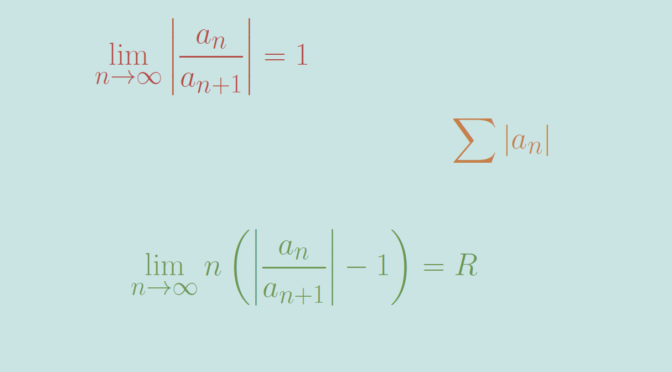# Raabe-Duhamel’s test

The Raabe-Duhamel’s test (also named Raabe’s test) is a test for the convergence of a series $\sum_{n=1}^\infty a_n$ where each term is a real or complex number. The Raabe-Duhamel’s test was developed by Swiss mathematician Joseph Ludwig Raabe.

It states that if:

$\displaystyle \lim _{n\to \infty }\left\vert{\frac {a_{n}}{a_{n+1}}}\right\vert=1 \text{ and } \lim _{{n\to \infty }} n \left(\left\vert{\frac {a_{n}}{a_{{n+1}}}}\right\vert-1 \right)=R,$
then the series will be absolutely convergent if $$R > 1$$ and divergent if $$R < 1$$. First one can notice that Raabe-Duhamel's test maybe conclusive in cases where ratio test isn't. For instance, consider a real $$\alpha$$ and the series $$u_n=\frac{1}{n^\alpha}$$. We have $\lim _{n\to \infty } \frac{u_{n+1}}{u_n} = \lim _{n\to \infty } \left(\frac{n}{n+1} \right)^\alpha = 1$ and therefore the ratio test is inconclusive. However $\frac{u_n}{u_{n+1}} = \left(\frac{n+1}{n} \right)^\alpha = 1 + \frac{\alpha}{n} + o \left(\frac{1}{n}\right)$ for $$n$$ around $$\infty$$ and $\lim _{{n\to \infty }} n \left(\frac {u_{n}}{u_{{n+1}}}-1 \right)=\alpha.$ Raabe-Duhamel's test allows to conclude that the series $$\sum u_n$$ diverges for $$\alpha <1$$ and converges for $$\alpha > 1$$ as well known.

When $$R=1$$ in the Raabe’s test, the series can be convergent or divergent. For example, the series above $$u_n=\frac{1}{n^\alpha}$$ with $$\alpha=1$$ is the harmonic series which is divergent.

On the other hand, the series $$v_n=\frac{1}{n \log^2 n}$$ is convergent as can be proved using the integral test. Namely $0 \le \frac{1}{n \log^2 n} \le \int_{n-1}^n \frac{dt}{t \log^2 t} \text{ for } n \ge 3$ and $\int_2^\infty \frac{dt}{t \log^2 t} = \left[-\frac{1}{\log t} \right]_2^\infty = \frac{1}{\log 2}$ is convergent, while $\frac{v_n}{v_{n+1}} = 1 + \frac{1}{n} +\frac{2}{n \log n} + o \left(\frac{1}{n \log n}\right)$ for $$n$$ around $$\infty$$ and therefore $$R=1$$ in the Raabe-Duhamel’s test.